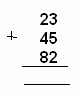Name: ___________________Date:___________________

 Email us to get an instant 20% discount on highly effective K-12 Math & English kwizNET Programs!

Method:
1. Arrange the numbers one after the other. Start from the ones place.
2. Add the three numbers in the ones place.
3. Add the three numbers in the tens place.
4. If there is a carry over, add it too.

Example:
23 + 45 + 82 =
 Step 1 Step 2 Step 3Directions: Find the sum of the 3 two digit numbers. Also write at least ten examples of your own.
 Q 1: Find the answer: 16 + 43 + 3 Answer: Q 2: Find the answer: 52 + 35 + 25 Answer: Q 3: Find the answer: 24 + 3 + 59 Answer: Q 4: Find the answer: 53 + 39 + 29 Answer: Q 5: Find the answer: 74 + 81 + 72 Answer: Question 6: This question is available to subscribers only! Question 7: This question is available to subscribers only! Question 8: This question is available to subscribers only! Question 9: This question is available to subscribers only! Question 10: This question is available to subscribers only!